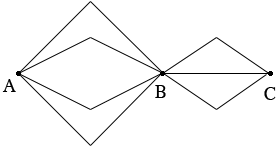SEARCH HOMEMath Central Quandaries & QueriesQuestion from Shawn: Hello, I am trying to solve a problem which is how many ways can you travel from A to D. A has 4 options, B has 3, C and you get to D. How many ways are there?Hi Shawn,

I drew a diagram of the ways to get from A to B and then from B to C.There are 4 roads from A to B and whichever one you choose you then have 3 roads to choose from to get to C. Hence there are $4 \times 3 = 12$ ways to get from A to C.

Now add D to the diagram. How many roads are there from C to D? You didn't say.

How many ways are there to get from A to D?

PennyMath Central is supported by the University of Regina and The Pacific Institute for the Mathematical Sciences.